# Additional problem 1 Let AABC be a triangle, let be the bisector of the angle ZCAB Let P be the intersection of and BC. Let R be the point on the line AB such that AR-AC, and let X-APnRC. Let Q denot...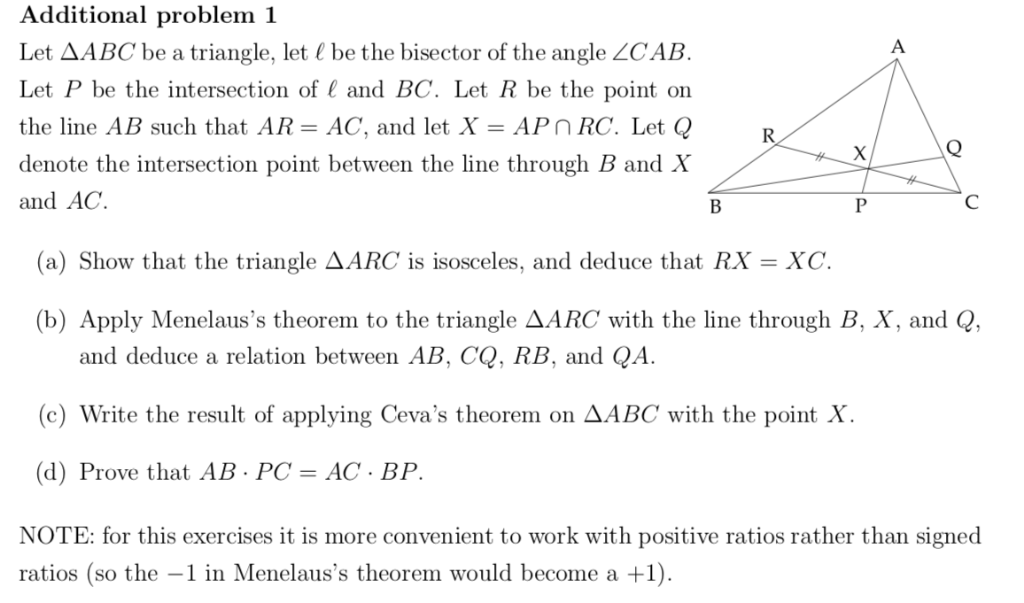Additional problem 1 Let AABC be a triangle, let be the bisector of the angle ZCAB Let P be the intersection of and BC. Let R be the point on the line AB such that AR-AC, and let X-APnRC. Let Q denote the intersection point between the line through B and X and AC. (a) Show that the triangle AARC is isosceles, and deduce that RX-XC. (b) Apply Menelaus's theorem to the triangle AARC with the line through B, X, and Q, and deduce a relation between AB, CQ, RB, and QA c) Write the result of applying Ceva's theorem on AABC with the point X (d) Prove that AB PC- AC BP NOTE: for this exercises it is more convenient to work with positive ratios rather than signed ratios (so the -1 in Menelaus's theorem would become a +1).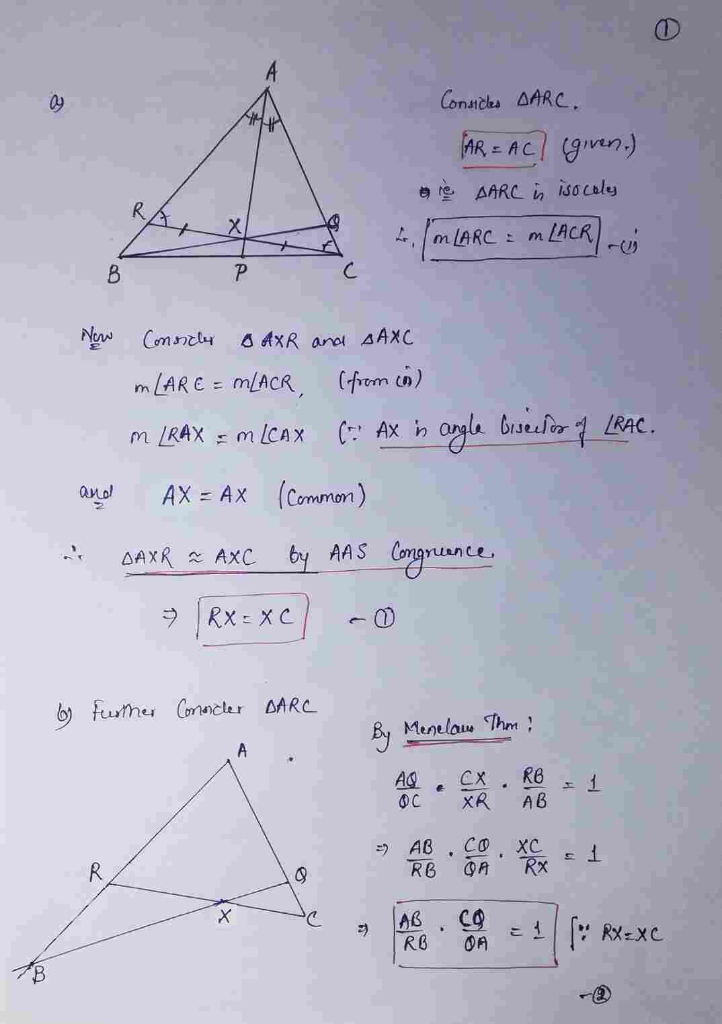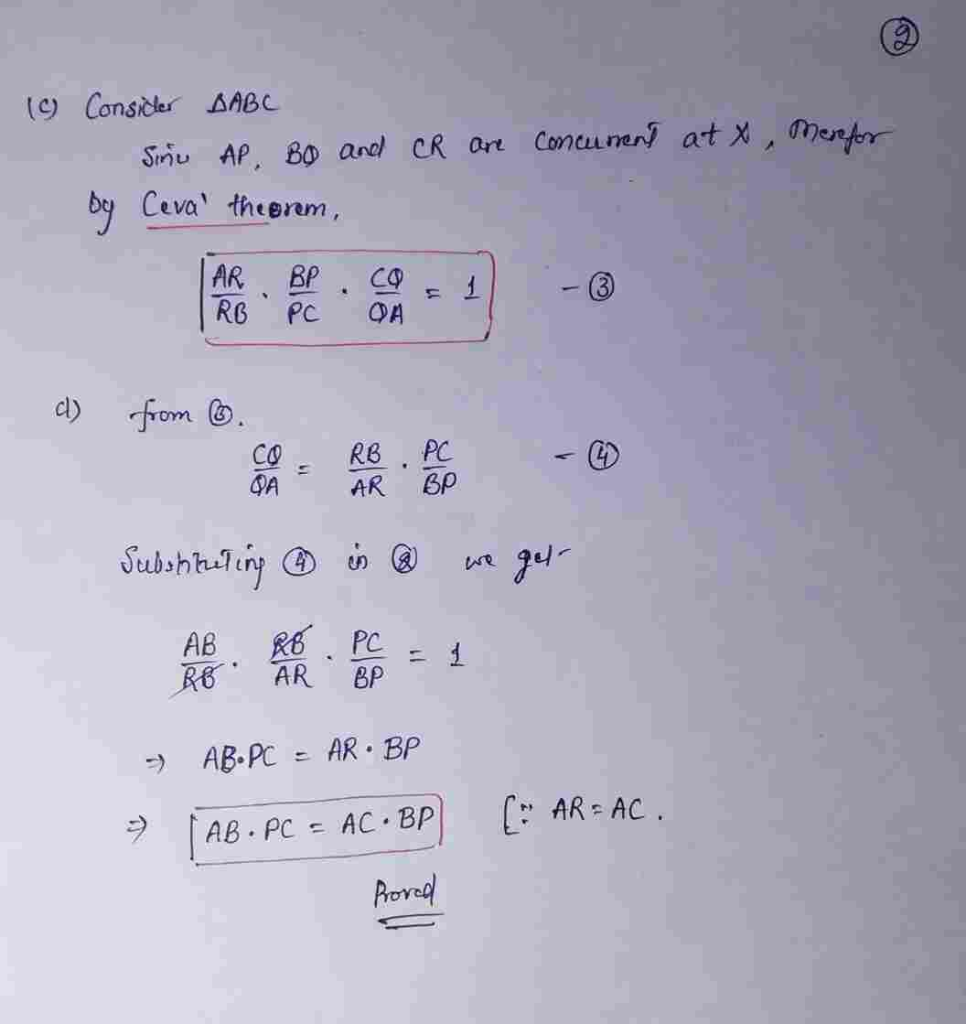##### Add Answer of: Additional problem 1 Let AABC be a triangle, let be the bisector of the angle ZCAB Let P be the intersection of and BC. Let R be the point on the line AB such that AR-AC, and let X-APnRC. Let Q denot...
Similar Homework Help Questions
• ### 520. Given triangle ABC, let F be the point where segment BC meets the bisector of angle BAC, Draw the line through B that is parallel to segment AF, and let E be the point where this parallel me...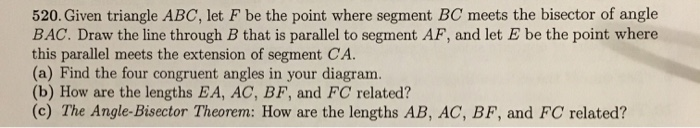520. Given triangle ABC, let F be the point where segment BC meets the bisector of angle BAC, Draw the line through B that is parallel to segment AF, and let E be the point where this parallel meets the extension of segment CA. (a) Find the four congruent angles in your diagram. (b) How are the lengths EA, AC, BF, and FC related? (c) The Angle-Bisector Theorem: How are the lengths AB, AC, BF, and FC related? 520. Given...

• ### Let P and Q be the points on the sides AB and BC of a triangle ABC respectively such that BP = 3PA and QC = 2BQ

Let P and Q be the points on the sides AB and BC of a triangle ABC respectively such that BP = 3PA and QC = 2BQ. Let K be the midpoint of the segment PQ. Prove that the area of the triangle AKC is equal to 11S/24, where S is the area of the triangle ABC.

• ### given isosceles triangle ABC, line AB = BC, and BD is the angle bisector of isosceles triangle ABC prove AB x DC = BC x AD Aren't the two halves congruent

given isosceles triangle ABC, line AB = BC, and BD is the angle bisector of isosceles triangle ABC prove AB x DC = BC x ADAren't the two halves congruent? (SAS) If so, then DC is equal to AD.Don't the ratios AB:AD=BC:DC by corresponding parts?how do i write that out like on a table though like step by stepI won't do the table otherwise that defeats the purpose of the assignment. If you do the exercise and post your work...

• ### In triangle ABC, angle C is congruent to angle A, line BC=2x-5, line AB=x+5 and line AC=x+10

In triangle ABC, angle C is congruent to angle A, line BC=2x-5, line AB=x+5 and line AC=x+10. Find x.The problem didn't have a triangle drawn so i drew it myself. I tried many equations but when i substitute it it didn't work.

• ### Let triangle ABC be a triangle such that angle ACB is 135 degrees

1. Let triangle ABC be a triangle such that angle ACB is 135 degrees.Prove that AB^2 = AC^2 + BC^2 - (Root 2) x AC x BC2. Simplify:2/1! - 3/2! + 4/3! - 5/4! + ..... + 2010/2009! - 2011/2010!Where k! is the multiplication of all numbers up to k (e.g. 5! = 5x4x3x2x1=120)3. For the algebra nerds:Factorise:a^4 + b^4 + c^2 - 2 (a^2 x b^2 + a^2 x c + b^2 x c)

• ### Triangle problemPROBLEM:You have a triangle ABC.The bisector BN is intesectedby the median AM at the point P.Draw line CP and extend itto AB, this intersection point islabeled Q.Now show thattriangle BNQ is isoceles.MY THOUGHTS:Ok, here's what I know so far... The drawing above is alreadylabeled and marked according to what I know (typed below), this isnot the drawing that comes from theoriginal problem.I don't know where the congruent symbol is, so I'm using an =sign.Also, sorry for the bad drawing.AN=NC, because...

• ### triangle ABC, AB = x cm, BC = (x-4)cm, AC =10 cm and angle BAC = 60° calclulate the value of x

triangle ABC, AB = x cm, BC = (x-4)cm, AC =10 cm and angle BAC = 60° calclulate the value of x

• ### 16) AB = 3.2cm BC = 8.4cm The area of the triangle ABC is 10cm²

16) AB = 3.2cm BC = 8.4cm The area of the triangle ABC is 10cm². Calculate the perimeter and give the answer correct to 3 significant figures. 19) Bill said that the line y=6 cuts the curve x² + y² =25 at two points. a) By eliminating y, show that Bill is incorrect. b) By eliminating y, find the solutions to the simultaneous equations x² + y² = 25 y = 2x - 2 26) c) Factorise (p+q)² + 5(p+q)...

• ### Let P be the point (p,q) and L the line y = m x + b

Let P be the point (p,q) and L the line y = m x + b. It is not necessary, but if you like you may assume that P lies below the line L. All your answers below should be algebraic expressions in terms of m, b, p and q. The slope of L is . The slope of a line perpendicular to L is . The line through P perpendicular to L can be written as y = s...

• ### 23.4 Let ABC be any triangle, let DE be a line parallel to the base, and let F be any point on DE. Show that the area of the union of the two triangles DBF and ECF is less than or equal to one-fourth...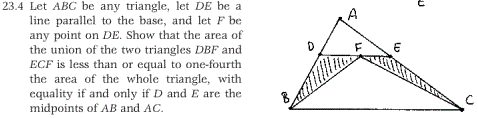23.4 Let ABC be any triangle, let DE be a line parallel to the base, and let F be any point on DE. Show that the area of the union of the two triangles DBF and ECF is less than or equal to one-fourth the area of the whole triangle, with equality if and only if D and E are the midpoints of AB and AC. 23.4 Let ABC be any triangle, let DE be a line parallel to the...

Need Online Homework Help?